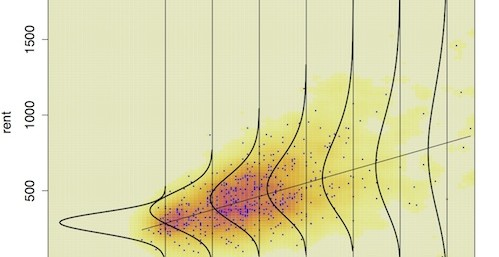## Overview

In the previous tutorials we covered how the multilevel model is used to examine intraindividual covariability. In this tutorial, we outline how an extension, the multilevel model with heterogeneous variance can be used to examine differences in intraindividual variability - which we had previously done in a 2-step way using the iSD.

## Outline

1. Introduction to The Variance Heterogeneity Model
2. A Series of Analysis Models
3. Empirical Examples
4. Conclusion

library(psych)
library(ggplot2)
library(nlme)

## 1. Introduction to the Variance Heterogeneity Model

We have used two “separate” sets of methods to examine …
1. Intraindividual Variation (calculation of within-person summaries; iSD, iEntropy, iMSSD, etc. following Ram & Gerstorf, 2009)
2. Intraindividual Covariation (multilevel models - following Bolger & Laurenceau, 2013)

In 1., our interest was in the interindividual differences in within-person variability in an outcome variable, $$\sigma_{yi}^2$$. In 2., our interest was in the interindividual differences in the within-person association between a predictor and outcome $$\beta_{1i}$$.

Here we outline a framework where these two interests can be combined together into a single model …. Multilevel Model with Heterogeneous Variance.

Like with multilevel modeling itself, the framework is referred to by different names in different fields. The larger class of models includes models referred to as … Variance Heterogeneity models, Location-Scale models, Generalized Additive Models for Location, Scale and Shape (GAMLSS, http://www.gamlss.org). It is not totally clear if there are some nuanced differences among the various different models. However, as far as I can tell, all of these model variants are applied to longitudinal panel-type data (multiple persons, multiple occassions).

A parallel form of the model exists for time-series data, ARCH models (auto-regressive models with conditional heteroscedasticity; https://en.wikipedia.org/wiki/Autoregressive_conditional_heteroskedasticity). There are lots of variants there including GARCH, NGARCH, IGARCH, EGARCH, GARCH-M, QGARCH, TGARCH. All of these model variants are applied to time-series data (single entity, many many occassions). I am under the impression that not many people have attempted to form links between these two different traditions, but have not yet looked into the literature closely.

Much of what we explore today is covered in Hedeker, Mermelstein, Berbaum, & Campbell (2009), Hedeker & Mermelstein (2007), and Hoffman (2007). Some more recent extensions are in Hedeker, Mermelstein & Demirtas (2012), and Rast, Hofer, & Sparks (2012).

#### Some Basic Definitions

A few definitions from Wikipedia (which may or may not be useful today, but are part of our larger effort to understand how we can make use of all these models).

Location parameter: https://en.wikipedia.org/wiki/Location_parameter
In statistics, a location family is a class of probability distributions that is parametrized by a scalar- or vector-valued parameter $$x_0$$, which determines the “location” or shift of the distribution. Examples of location parameters include the mean, the median, and the mode.

Scale parameters: https://en.wikipedia.org/wiki/Scale_parameter
In probability theory and statistics, a scale parameter is a special kind of numerical parameter of a parametric family of probability distributions. The larger the scale parameter, the more spread out the distribution.

The normal distribution has two parameters: a location parameter $$\mu$$ and a scale parameter $$\sigma$$. In practice the normal distribution is often parameterized in terms of the squared scale $$\sigma^2$$, which corresponds to the variance of the distribution.

Here you can see location and scale of the distribution changing over time (image from http://www.gamlss.org)Shape parameters: https://en.wikipedia.org/wiki/Shape_parameter
A shape parameter is any parameter of a probability distribution that is neither a location parameter nor a scale parameter (nor a function of either or both of these only, such as a rate parameter). Such a parameter must affect the shape of a distribution rather than simply shifting it (as a location parameter does) or stretching/shrinking it (as a scale parameter does). Distributions with a shape parameter include skew normal, gamma, Weibull, ExGaussian distributions.

#### The Usual (Homogeneous Variance) Multilevel Model

Typically, the multilevel models we use (and that are covered in B&L) make a homogeneity of variance assumption. For example, lets look at the basic “unconditional means” model.

$y_{it} = \beta_{0i} + e_{it}$ $\beta_{0i} = \gamma_{00} + u_{0i}$

where $\mathbf{e_{it}} \sim \mathcal{N}(0,\mathbf{R})$, and $\mathbf{u_{i}} \sim \mathcal{N}(0,\mathbf{G})$.

For clarity, lets write out the full variance covariance matrix of the between-person residuals, $$\mathbf{G}$$. In this case it is just a 1 x 1 matrix. $\mathbf{G} = \left[\begin{array} {r} \sigma^{2}_{u0} \end{array}\right]$ Embedded here is the assumption that the between-person variance characterizes the entire sample (population). There are no subgroups. Later, we will relax this assumption, and allow that $$\sigma^{2}_{u0g}$$ can differ across “groups” of individuals (e.g., Males, Females).

For clarity, lets also write out the full variance covariance matrix of the within-person residuals, $$\mathbf{R}$$ (spanning across the t = 8 days of observation in the example data), $\mathbf{R} = \mathbf{I} \left[\begin{array} {r} \sigma^{2}_{e} \end{array}\right] = \left[\begin{array} {rrrrrrrr} \sigma^{2}_{e} & 0 & 0 & 0 & 0 & 0 & 0 & 0 \\ 0 & \sigma^{2}_{e} & 0 & 0 & 0 & 0 & 0 & 0 \\ 0 & 0 & \sigma^{2}_{e} & 0 & 0 & 0 & 0 & 0 \\ 0 & 0 & 0 & \sigma^{2}_{e} & 0 & 0 & 0 & 0 \\ 0 & 0 & 0 & 0 & \sigma^{2}_{e} & 0 & 0 & 0 \\ 0 & 0 & 0 & 0 & 0 & \sigma^{2}_{e} & 0 & 0 \\ 0 & 0 & 0 & 0 & 0 & 0 & \sigma^{2}_{e} & 0 \\ 0 & 0 & 0 & 0 & 0 & 0 & 0 & \sigma^{2}_{e} \end{array}\right]$, The homoscedasticity of errors applies across persons and occasions. There are no subgroups. Later, we will relax this assumption, and allow that $$\sigma^{2}_{eg}$$ can differ across g “groups” of individuals (e.g., males, females).

Here is a little plot of some simulated data that follow this model.